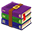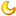# 51单片机输出8路脉冲Proteus仿真+程序0 |0 | 2020-1-22 12:34:07 | 显示全部楼层 |阅读模式#include #define unit unsigned int #define uchar unsigned char sbit p10=P1^0;//P1^0输出100HZ波形 sbit p11=P1^1;//P1^1输出50HZ波形 sbit p12=P1^2;//P1^2输出25HZ波形 sbit p13=P1^3;//P1^3输出20HZ波形 sbit p14=P1^4;//P1^4输出10HZ波形 sbit p15=P1^5;//P1^5输出5HZ波形 sbit p16=P1^6;//P1^6输出2HZ波形 sbit p17=P1^7;//P1^7输出1HZ波形 uchar count0;//计数 uchar count1; uchar count2; uchar count3; uchar count4; uchar count5; uchar count6; uchar count7; void main() {         count0=1;         count1=2;         count2=4;         count3=5;         count4=10;         count5=20;         count6=50;         count7=100;         TMOD=0X01;//定时器0 方式1         TH0=(65536-5000)/256;//5000个机器周期  5ms         TL0=(65536-5000)%256;         EA=1;         ET0=1;         TR0=1;         while(1)         {                 if(count0==0){count0=1;p10=!p10;}                 if(count1==0){count1=2;p11=!p11;}                 if(count2==0){count2=4;p12=!p12;}                 if(count3==0){count3=5;p13=!p13;}                 if(count4==0){count4=10;p14=!p14;}                 if(count5==0){count5=20;p15=!p15;}                 if(count6==0){count6=50;p16=!p16;}                 if(count7==0){count7=100;p17=!p17;}         } } void counter()interrupt 1 {          TH0=(65536-4994)/256;         TL0=(65536-4994)%256;         count0--;         count1--;         count2--;         count3--;         count4--;         count5--;         count6--;         count7--; }复制代码51单片机输出8路脉冲Proteus仿真 程序.rar (27.78 KB, 售价: 1 工控币) 2020-1-22 12:33 上传 点击文件名下载附件

 本版积分规则 回帖后跳转到最后一页425主题 346积分346